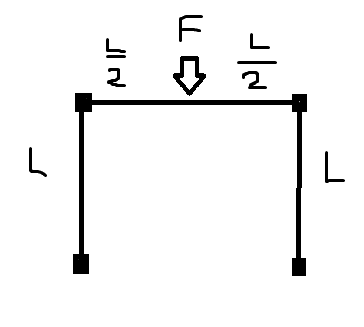# Bending Moment Diagram

axe34

## Homework StatementHi I need help with drawing a bending moment diagram for this structure. All joints are rigid and it's fixed to the ground. I'm really struggling with this. I initially started with the horizontal beam (statically indeterminate) and found that the moments at the ends were FL/8 (ccw on left, and cw on right) and that F/2 was applied up at each end of the beam. However, this is not the correct answer! Also this answer doesn't give the correct answer for the vertical beams. I really don't know how to do this.

## Homework Equations

d2v/ dx2 = -M (helps with statically indeterminate beams but this is not the only way to deal with them but I like this method and this is how I calculated the FL/8's)

as above

## Answers and Replies

paisiello2
Your equation isn't complete.

Can you show your work on how you derived FL/8?

axe34
Hi

For a fixed-fixed beam only, there are BMs of FL/8 - this is a standard result. It would take pages + pages to show this, but it starts from the fact that d2v/dx2 = -M. Fl/8 isn't correct here in this structure.

paisiello2
You are right the beam is not fixed-fixed but your equation is not quite correct.

Do you know the stiffness matrix for a beam element?

axe34
Hi
I know nothing about stiffness etc. - only each beam is L length and the same as the others.

paisiello2
Generally the bending moment will be a function of the members' stiffness for a statically indeterminate frame. If all the members have the same stiffness (EI) then the term simply cancels out.

So you can use this method or you could do a moment distribution method. Have you studied either of these methods?

axe34
Hi
The only method I've ever used for 1 statically indeterminate beam is to do EI * d2v/dx2 = -M. I just don't know where to start on this one. If I was given one horizontal beam, fixed at both ends, then using this method I'd get moments of F*L /8 at the ends

paisiello2
OK, this will be a long process starting from such a basic formula.

The first step I would recommend is to simplify the problem using the symmetry of the frame and leave just two degrees of freedom.

The 2nd step I would recommend is to derive two equations from these two degrees of freedom.

Do you happen to know any formulae that cover the following conditions:

1) for beam fixed-pinned with a moment M at the pinned end - formula for dv/dx at pinned end?
2) for beam fixed-fixed with a displacement v at one end - formula for M at ends?